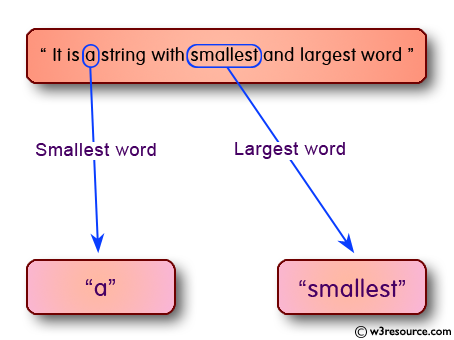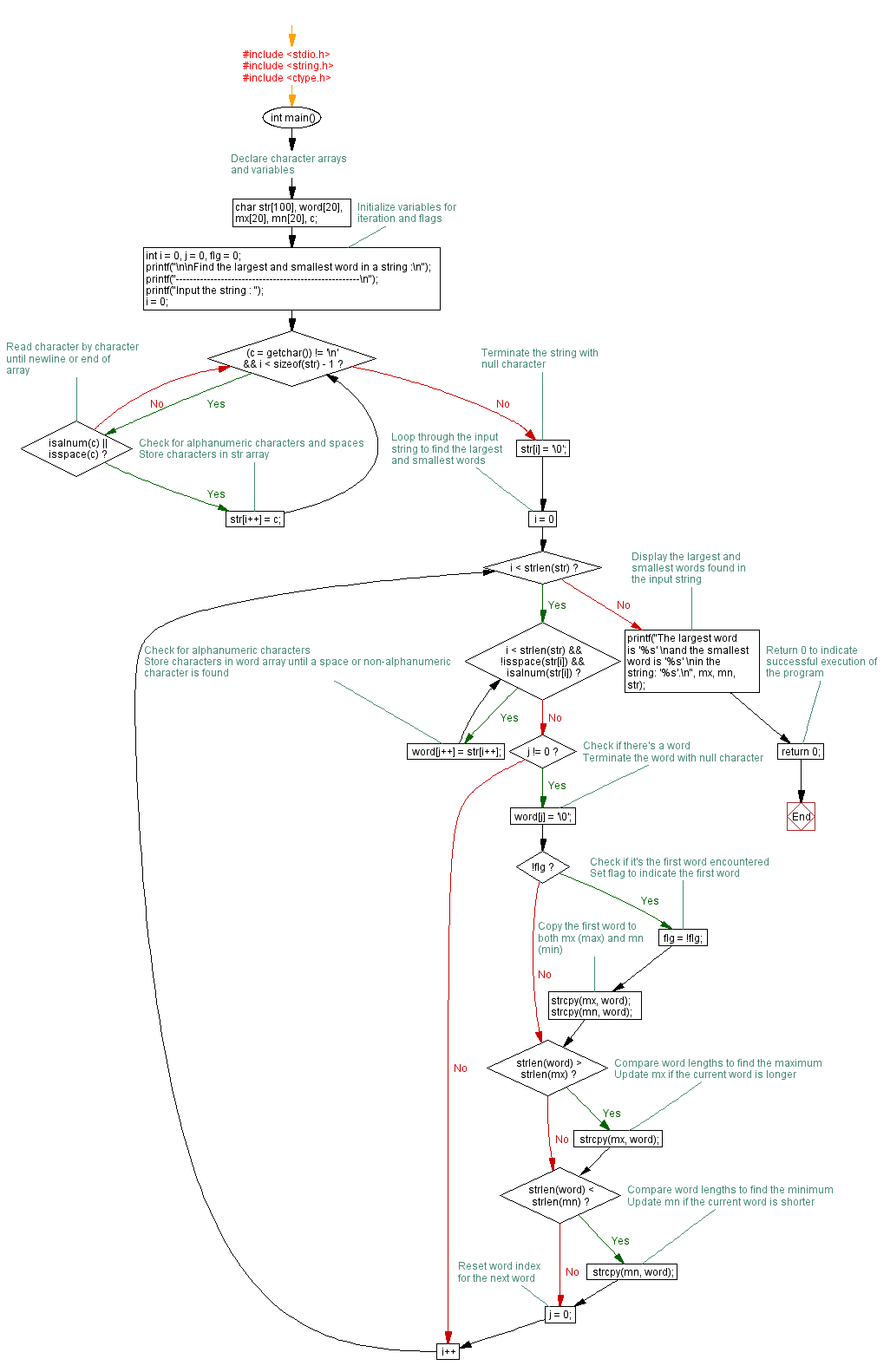﻿ C exercises: Find the largest and smallest word in a string - w3resource# C Programming: Find the largest and smallest word in a string

## C String: Exercise-20 with Solution

Write a program in C to find the largest and smallest word in a string.Sample Solution:

C Code:

``````#include <stdio.h>
#include <string.h>
#include <ctype.h>

void main()
{
char str, word, mx, mn, c;
int i = 0, j = 0, flg = 0;

printf("\n\nFind the largest and  smallest word in a string :\n");
printf("-----------------------------------------------------\n");

printf("Input the string : ");
i = 0;
do
{
fflush(stdin);
c = getchar();
str[i++] = c;

} while (c != '\n');
str[i - 1] = '\0';
for (i = 0; i < strlen(str); i++)
{
while (i < strlen(str) && !isspace(str[i]) && isalnum(str[i]))
{
word[j++] = str[i++];
}
if (j != 0)
{
word[j] = '\0';
if (!flg)
{
flg = !flg;
strcpy(mx, word);
strcpy(mn, word);
}
if (strlen(word) > strlen(mx))
{
strcpy(mx, word);
}
if (strlen(word) < strlen(mn))
{
strcpy(mn, word);
}
j = 0;
}
}
printf("The largest word is '%s' \nand the smallest word is '%s' \nin the string : '%s'.\n", mx, mn, str);
}
```
```

Sample Output:

```Find the largest and  smallest word in a string :
-----------------------------------------------------
Input the string : It is a string with smallest and largest word.
The largest word is 'smallest'
and the smallest word is 'a'
in the string : 'It is a string with smallest and largest word'.
```

Flowchart :C Programming Code Editor:

Improve this sample solution and post your code through Disqus.

What is the difficulty level of this exercise?

﻿

## C Programming: Tips of the Day

Is there a way to specify how many characters of a string to print out using printf()?

The basic way is:

`printf ("Here are the first 8 chars: %.8s\n", "A string that is more than 8 chars"); `

The other, often more useful, way is:

`printf ("Here are the first %d chars: %.*s\n", 8, 8, "A string that is more than 8 chars"); `

Here, you specify the length as an int argument to printf(), which treats the '*' in the format as a request to get the length from an argument.

You can also use the notation:

```printf ("Here are the first 8 chars: %*.*s\n",
8, 8, "A string that is more than 8 chars"); ```

This is also analogous to the "%8.8s" notation, but again allows you to specify the minimum and maximum lengths at runtime - more realistically in a scenario like:

`printf("Data: %*.*s Other info: %d\n", minlen, maxlen, string, info); `

The POSIX specification for printf() defines these mechanisms.

Ref: https://bit.ly/395Zj2j